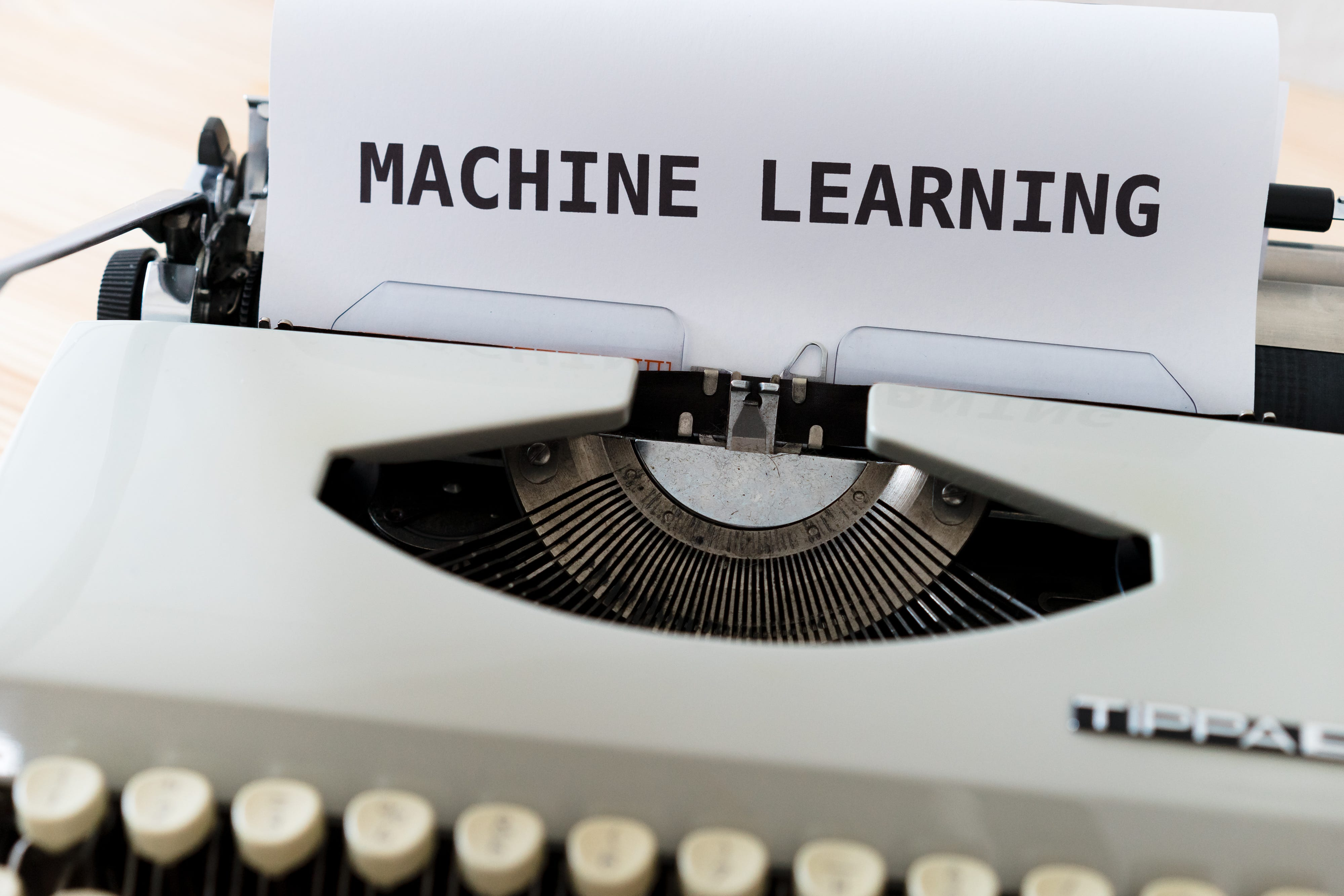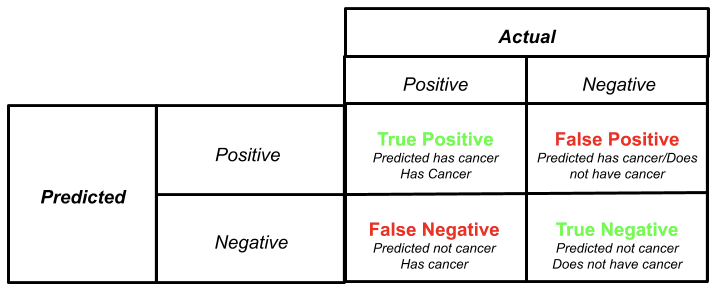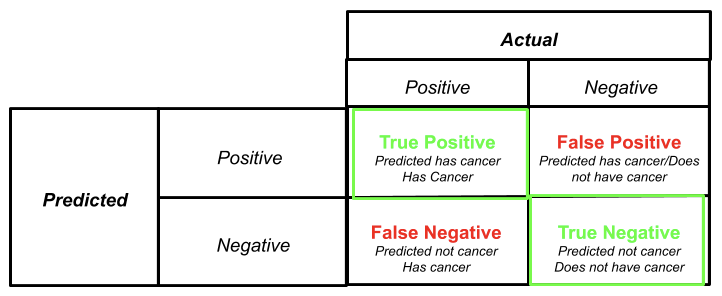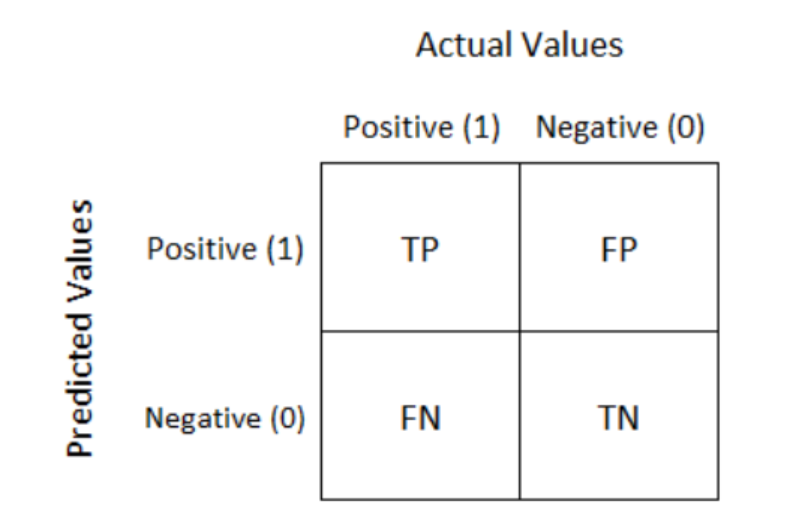1626121740

# Classification Metrics

In the regular world, accuracy and precision are often interchangeable — but not when it comes to machine learning. Accuracy and precision are really important metrics that are used for model evaluation, and together with recall and F1, they make up the famous classification metrics.

confusion matrix is the best tool that can be used to completely understand why these four metrics are so important for model evaluation. Here is what it looks like:

If you are confused, don’t worry. It’s normal. This matrix is really two tables merged into one — table showing the predicted values, and another table showing the actual values. The result of merging the two tables are True Positives, True Negatives, False Positives, and False Negatives. Here is what each of them means:

1. True Positive (TP): Predicted value and actual values are positive.
2. True Negatives (TN): Predicted value and actual values are negative.
3. False Negative (FN): Actual value is positive but the predicted value is negative.
4. False Positive (FP): Actual value is negative but the predicted value is positive.

As you can see, this matrix helps to not only measure the performance of a predictive model, but also shows insight into which classes are being predicted incorrectly or correctly, and where the errors are occurring. Now that we understand a bit about the confusion matrix, let’s look at how it helps to define the classification metrics.

## F1 Score

#machine-learning #classification-metrics #scikit-learn #metrics

## Buddha Community1626121740

## Classification Metrics

In the regular world, accuracy and precision are often interchangeable — but not when it comes to machine learning. Accuracy and precision are really important metrics that are used for model evaluation, and together with recall and F1, they make up the famous classification metrics.

confusion matrix is the best tool that can be used to completely understand why these four metrics are so important for model evaluation. Here is what it looks like:

If you are confused, don’t worry. It’s normal. This matrix is really two tables merged into one — table showing the predicted values, and another table showing the actual values. The result of merging the two tables are True Positives, True Negatives, False Positives, and False Negatives. Here is what each of them means:

1. True Positive (TP): Predicted value and actual values are positive.
2. True Negatives (TN): Predicted value and actual values are negative.
3. False Negative (FN): Actual value is positive but the predicted value is negative.
4. False Positive (FP): Actual value is negative but the predicted value is positive.

As you can see, this matrix helps to not only measure the performance of a predictive model, but also shows insight into which classes are being predicted incorrectly or correctly, and where the errors are occurring. Now that we understand a bit about the confusion matrix, let’s look at how it helps to define the classification metrics.

## F1 Score

#machine-learning #classification-metrics #scikit-learn #metrics1597252500

## Classification Metrics & Thresholds Explained

### Demystifying commonly used classification metricsPhoto by Markus Winkler on Unsplash

## Classification Evaluation

The metric we use to evaluate our classifier depends on the nature of the problem we want to solve and the potential consequences of prediction error. Let’s examine a very common example of cancer diagnosis (ie. classified as having cancer or not having cancer). We want our model to predict as many actual/true cancer diagnoses as possible but we also know that it is statistically impossible to correctly identify all true cancer diagnoses. Our model will eventually classify/predict someone to have cancer when they actually don’t have cancer (false positive) and predict someone not to have cancer when they actually have cancer (false negative). The question we have to ask ourselves is “What is worse? Predicting someone to have cancer when they actually don’t or predicting someone not to have cancer when they do?”. The answer in this example is obvious as the consequences of telling someone they don’t have cancer when they do far outweigh the former. Let’s keep this example in mind but let’s review the commonly used classification performance metrics.

### Confusion MatrixA confusion matrix summarizes are the model’s predictions. It gives us the number of correct predictions (True Positives and True Negatives) and the number of incorrect predictions (False Positives and False Negatives). In our cancer example, if our model predicted someone to have cancer and the person has cancer that’s a true positive. When our model predicted someone not to have cancer and that person does not have cancer that’s a true negative. When our model predicted someone to have cancer but that person does not have cancer that’s a false positive (ie. the model falsely predicted a positive cancer diagnosis). Finally, when our model predicted someone not to have cancer but they do that’s a false negative (ie. the model falsely predicted a negative cancer diagnosis).

Much of the remaining performance metrics are derived from the confusion matrix therefore, it is imperative you have a good understand.

### Accuracy

In simplest terms, accuracy details how often our model is correct. In other words, is the number of correct predictions (TP, TF) divided by the total number of predictions. Accuracy is typically the first metric but it can be very misleading if not considered carefully. For example, let’s consider an imbalanced dataset that was used to train our model. We have 1000 non-cancer diagnoses and 10 cancer diagnoses. A model was able to correctly predict 900 of the non-cancer diagnoses and 1 of the cancer diagnoses would have an accuracy of 0.89% ((900+1)/1010=0.89).

(TP+TN)/(TP+FP+FN+TN)#precision-recall #roc-curve #f1-score #classification-metrics #python1592395200

## Still using Accuracy as a Classification Metric?

Accuracy is the most common evaluation metric for classification models because of its simplicity and interpretation. But when you have a multiclass classification problem in hand, say, for example, with 15 different target classes, looking at the standard accuracy of the model might be misleading. This is where “top N” accuracies might be of some use, and in this post, I’ll take you through the basic intuition and python implementation of top N accuracies.
Before we get into top N accuracy, a small refresher on standard accuracy metric:

#python #evaluation-metric #accuracy #machine-learning #classification-algorithms1599556620

## Evaluation Metrics for Your Machine Learning Classification Models

The most important part of any Machine Learning Model is to know how good or accurate your model is. Okay, so I am a budding Data Scientist and I start building models. But, how do I know the model that I built is good enough. You need to have certain parameters that will define the quality of the model. Isn’t it? Evaluating the quality of the model is very important in improving the model until it performs the best.

So, when it comes to Classification models, the evaluation metrics compare both expected and predicted output to come up with the probability for the class labels. Let’s just understand what is Classification problem. These are the ones where you can clearly see the target variable is divided into classes. If it can be divided into two classes, then it is called Binary Classification Problem and if you can divide it into more than 2 classes then it is called Multi-Class Classification Problem.

So, moving ahead with Evaluation Metrics for Classification Models. Below listed are the metrics used and we will discuss one by one.

1- Accuracy (Not in Case of Imbalanced Classes).

2- Confusion Matrix.

3- Precision.

4- Recall.

5- F1 Score.

6- AUC/ROC.

Let us understand further.

Accuracy:

Okay, let us get this straight into our minds. By Accuracy, what we mean is Classification Accuracy. So, it can be defined as the ration of ‘Number of Correct Prediction’ to Total prediction/Total number of input samples.

Let’s just say we had 5 input out of which we predicted 4 to be correct. Then,

Accuracy = 4/5 = 0.8 = 80%.

Accuracy is one of the simplest Metrics to be used. But, is it the best metrics? Well, the answer is a big NO. Let’s find out why with an example.

Let’s assume that we building a model to predict whether the transaction is fraudulent or not. Well, we built a model with an accuracy 99%. Why is accuracy such high, well it’s because of the class imbalance. Most of the transactions would not be fraudulent. So, if you fit a model that predicts the transaction to be not fraudulent, the accuracy remains 99% owing to class imbalance. Because of the class imbalance, the accuracy shoots up and is not the correct metrics to be used.

#evaluation-metric #data-science #classification-models #machine-learning #confusion-matrix1595851380

## Performance Metrics for Classification Machine Learning Problems

Many learning algorithms have been proposed. It is often valuable to assess the efficacy of an algorithm. In many cases, such assessment is relative, that is, evaluating which of several alternative algorithms is best suited to a specific application.

People even end up creating metrics that suit the application. In this article, we will see some of the most common metrics in a classification setting of a problem.

The most commonly used Performance metrics for classification problem are as follows,

• Accuracy
• Confusion Matrix
• Precision, Recall, and F1 score
• ROC AUC
• Log-loss

# Accuracy

Accuracy is the simple ratio between the number of correctly classified points to the total number of points.

To calculate accuracy, scikit-learn provides a utility function.

``````from sklearn.metrics import accuracy_score

#predicted y values
y_pred = [0, 2, 1, 3]
#actual y values
y_true = [0, 1, 2, 3]
accuracy_score(y_true, y_pred)
0.5
``````

Accuracy is simple to calculate but has its own disadvantages.

## Limitations of accuracy

• If the data set is highly imbalanced, and the model classifies all the data points as the majority class data points, the accuracy will be high. This makes accuracy not a reliable performance metric for imbalanced data.
• From accuracy, the probability of the predictions of the model can be derived. So from accuracy, we can not measure how good the predictions of the model are.

# Confusion Matrix

Confusion Matrix is a summary of predicted results in specific table layout that allows visualization of the performance measure of the machine learning model for a binary classification problem (2 classes) or multi-class classification problem (more than 2 classes)Confusion matrix of a binary classification

• TP means True Positive. It can be interpreted as the model predicted positive class and it is True.
• FP means False Positive. It can be interpreted as the model predicted positive class but it is False.
• FN means False Negative. It can be interpreted as the model predicted negative class but it is False.
• TN means True Negative. It can be interpreted as the model predicted negative class and it is True.

For a sensible model, the principal diagonal element values will be high and the off-diagonal element values will be below i.e., TP, TN will be high.

To get an appropriate example in a real-world problem, consider a diagnostic test that seeks to determine whether a person has a certain disease. A false positive in this case occurs when the person tests positive but does not actually have the disease. A false negative, on the other hand, occurs when the person tests negative, suggesting they are healthy when they actually do have the disease.

For a multi-class classification problem, with ‘c’ class labels, the confusion matrix will be a (c*c) matrix.

To calculate confusion matrix, sklearn provides a utility function

#data-science #beginners-guide #machine-learning #performance-metrics #classification-algorithms #deep learning